• 本代码主要利用MATLAB工具实现MATLAB 矩阵元素的区域访问，简单明了，易于理解
• MATLAB对矩阵中元素的访问

万次阅读 2019-03-04 15:18:18
MATLAB矩阵的建立与访问 矩阵访问一律用()，元胞数组用{} a(i,j)%第i行第j列队元素 a(:,j)%第j列的所有元素 a(2:end,j)%第j列中第2行到最后一行的元素 a(:,3:5)%第3〜5列的所有元素 a(x)%第x个元素（x为从最左边第1...

MATLAB矩阵的建立与访问
矩阵访问一律用()，元胞数组用{}
a(i,j)%第i行第j列队元素
a(:,j)%第j列的所有元素
a(2:end,j)%第j列中第2行到最后一行的元素
a(:,3:5)%第3〜5列的所有元素
a(x)%第x个元素（x为从最左边第1列开始编号）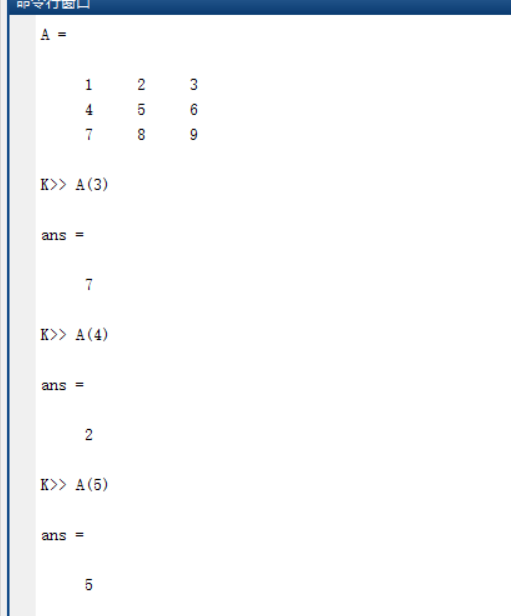展开全文• MATLAB矩阵进行多元素访问q=randn(4) q1=q(1,:) %访问第一行 q2=q(1:3,:) %访问1-3行 q3=q(:,2) %访问第二列 q4=q(:) %访问所以元素 运行结果如下

MATLAB对矩阵进行多元素访问

q=randn(4)
q1=q(1,:)          %访问第一行
q2=q(1:3,:)        %访1-3行
q3=q(:,2)          %访问第二列
q4=q(:)             %访问所以元素

运行结果如下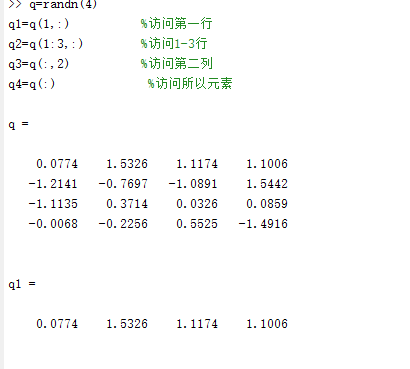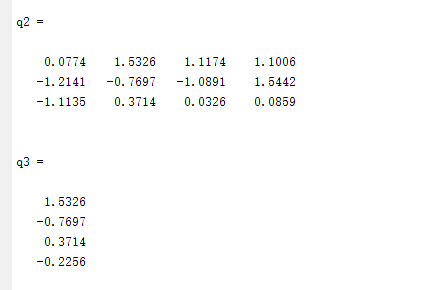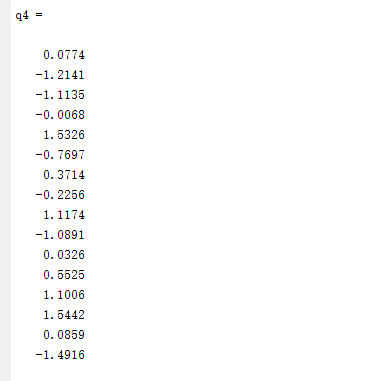展开全文matlab
• 【MATLAB】矩阵元素的引用

千次阅读 2020-07-14 21:25:11
一、矩阵元素的引用方式 1、通过下标来引用矩阵的元素，下标必须为正整数，且要用圆括号括起来 ...在MATLAB中，矩阵元素按列存储，即首先存储矩阵的第一列元素，然后存储第二列元素，…，一直到矩阵的

一、矩阵元素的引用方式

1、通过下标来引用矩阵的元素，下标必须为正整数，且要用圆括号括起来

例子（1）：
A(3,2)表示A矩阵第3行第2列的元素。
如果要给这个元素赋值200。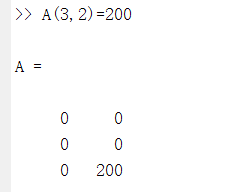注意：这只是改变了A（3,2）这个元素的值，并不会影响到其他的元素

例子（2）：

当给出的行列下标超出原来矩阵的行数和列数的时候，在matlab中将自动扩展原来的矩阵，并将扩展后没有赋值的矩阵元素，置为0.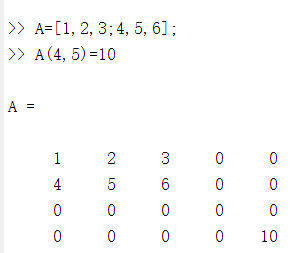2、通过序号来引用

在MATLAB中，矩阵元素按列存储，即首先存储矩阵的第一列元素，然后存储第二列元素，…，一直到矩阵的最后一列元素。

矩阵元素的序号就是矩阵元素在内存中的排列顺序。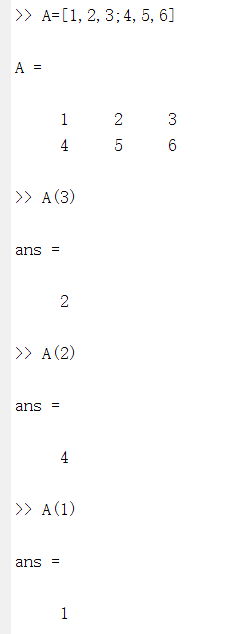序号与下标是一一对应的，以m×n矩阵A为例，矩阵元素A(i,j)的序号为(j-1)×m+i

矩阵元素的序号与下标可以利用sub2ind和ind2sub函数实现相互转换。
sub2ind函数：将矩阵中指定元素的行、列下标转换成存储的序号。调用格式为：
D=sub2ind(S,I,J)
D：表示对应下标元素的序号，它的行列数与I和J相同
S：表示要转换的的矩阵的行数和列数，是由行数和列数组成的向量，通常用size函数来获取
I：是要转化矩阵元素的行下标
J：是要转换矩阵的列下标
如果I和J是矩阵，表示要将矩阵中的多个元素的行列下标转换成存储的序号，及I和J的行列数必须相同。sub2ind函数中第一个参数通过size函数来获取，得到矩阵A的行数和列数，是一个包含两个元素的向量，分别为A矩阵的行数2和列数3。第二个和第三个参数都是用矩阵表示的，两个矩阵对应的元素代表矩阵A 中的某个元素的行下标和列下标，由调用结果可知：行下标为1，列下标为1的元素，在矩阵中是序号为1的；行下标为2列下标为1的元素在矩阵中序号为2；行下标为2列下标为3的元素在矩阵中序号为6；行下标为2列下标为2的元素在矩阵中序号为4.

ind2sub函数：将把矩阵元素的序号转换成对应的下标，其调用格式为：

[I,J]=ind2sub(S,D)

S：表示要转换的矩阵对的行数和列数，是由行数和列数组成的有两个元素的向量
D：序号，函数返回值为序号所对应元素的行下标和列下标
I：对应元素的行下标
j ；对应元素的列下标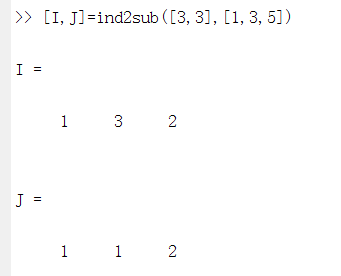[3,3]表示是一个3行3列的矩阵
[1,3,5]表示要求一个3行3列矩阵的第一个，第三个，第五个元素的下标
结果表明：第一个，第三个，第五个元素的下标分别为(1,1),(3,1),(2,2)。

二、利用冒号表达式获得子矩阵

子矩阵是指由矩阵中的一部分元素构成的矩阵。
A(i,:) 第i行的全部元素
A(:,j) 第j列的全部元素
A(i:i+m,k:k+m) 第i～i+m行内且在第k～k+m列中的所有元素
A(i:i+m,:) 第i～i+m行的全部元素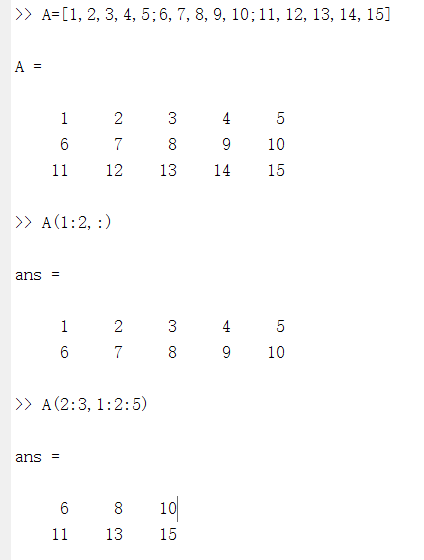end运算符：表示某一维的末尾元素下标。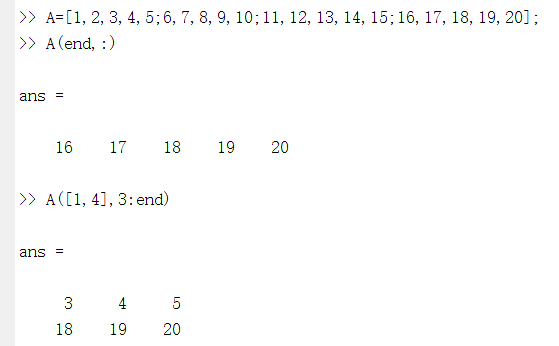A(end,:)表示应用矩阵A 的最后一行的元素。
A([1,4],3:end)表示应用第一行和第四行的从第三列到最后一列的元素。

三、利用空矩阵删除矩阵的元素

空矩阵是指没有任何元素的矩阵。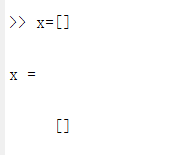X是一个空矩阵。如果要将某些元素从一个矩阵中删除，直接将这些元素置为空矩阵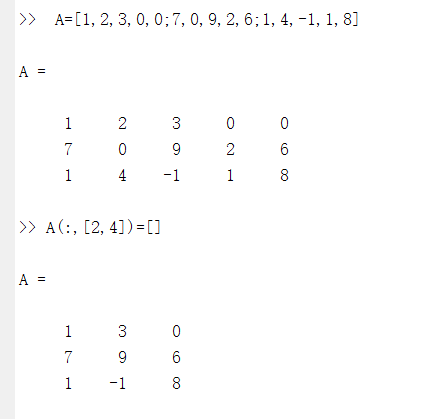A(:,[2,4])=[]表示赋空矩阵，删除第二列和第四列元素。

四、改变矩阵的形状

reshape(A,m,n)：在矩阵总元素保持不变的前提下，将矩阵A重新排成m×n的二维矩阵。
注意：reshape函数只是改变原矩阵的行数和列数，但并不改变原矩阵元素个数及其存储顺序。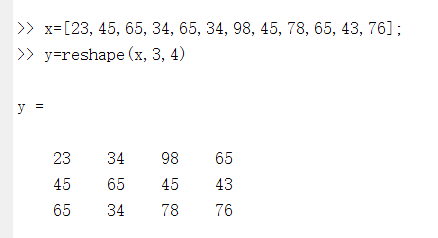y=reshape(x,3,4)表示调用reshape函数，利用向量x建立3行4列的矩阵赋给y
矩阵x为1行12列的矩阵
矩阵y为2行4列的矩阵

A(: ) ：将矩阵A的每一列元素堆叠起来，成为一个列向量。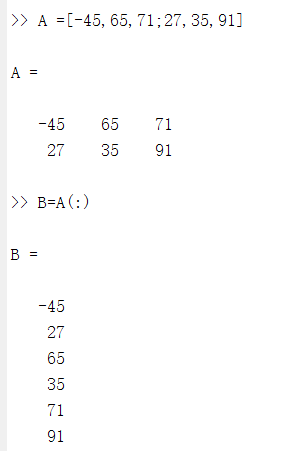可知A(:)等价于reshape(A,6,1)。

展开全文• 1、普通矩阵的建立不管是任何矩阵(向量)，都可以直接按照行方式输入每个元素：同一行的元素用逗号或者用空格符来分割，且空格个数不限；不同的行用分号分割。所有的元素都处于一方括号[]内。比如：>>Matrix_2D...

1、普通矩阵的建立

不管是任何矩阵(向量)，都可以直接按照行方式输入每个元素：同一行的元素用逗号或者用空格符来分割，且空格个数不限；不同的行用分号分割。所有的元素都处于一方括号[]内。比如：

>>Matrix_2D=[1,2,3;4,5,6;7,8,9]    %生成一个3×3的二维矩阵

用size() 函数可以计算一个矩阵的行数和列数。

2、创建三维数组的三种方法

(1)使用下标创建三维数组

在Matlab命令框中输入以下代码可以创建简单的三维数组：

for i=1:2

for j=1:2

for k=1:2

A(i,j,k)=i+j+k;

end

end

end

(2)使用低维数组创建三维数组

可以先创建一个二维数组，然后再通过第三维数组与其关系生成第三维数组；

D2=[1,2,3;4,5,6;7,8,9];

D3(:,:,1)=D2;

D3(:,:,2)=2*D2;

D3(:,:,3)=3*D2;

(3)使用创建函数创建三维数组

使用cat命令来创建高维数组。cat命令：C=cat(dim,A1,A2,A3,A4......)  其中dim表示的是创建数组的维度，A1,A2,A3,A4表示的是各维度上的数组。

D2=[1,2,3;4,5,6;7,8,9];

C=cat(3,D2,2*D2,3*D2);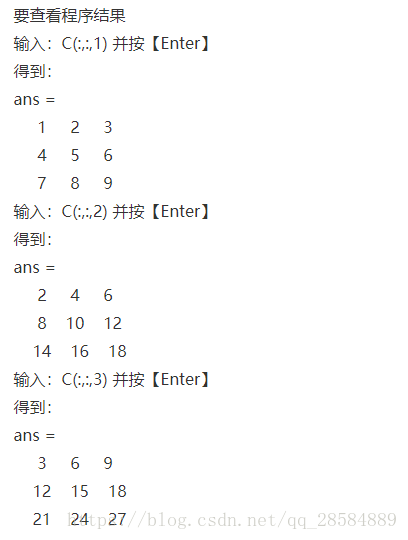3、矩阵的访问

访问矩阵中的元素有两种方式，一种是根据下标访问，另一种是根据序号访问。

以二维矩阵为例，根据下标访问时，将需要访问的矩阵元素的行列坐标写在圆括号内。比如：A(2,3)

可以使用“：”来访问所有坐标，或用end访问末尾坐标；例如：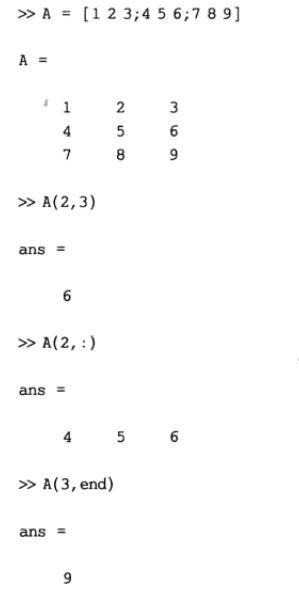矩阵访问一律用()，元胞数组用{}

a(i,j)%第i行第j列队元素

a(:,j)%第j列的所有元素

a(2:end,j)%第j列中第2行到最后一行的元素

a(:,3:5)%第3〜5列的所有元素

a(x)%第x个元素(x为从最左边第1列开始编号，若a为4行5列，则a(10)==a(2,3))

另一种是根据序号来访问矩阵元素，这种访问方式是将矩阵先转换为一维的向量，然后根据每个元素在该向量中的序号来访问元素，书写时将需要访问的元素序号写在圆括号内即可，如A(10)  ；  也可以使用“：”访问所有或者部分序号，用end访问末尾序号。在MATLAB中所有二维转换为一维的情况都是按照列优先的顺序来访问的。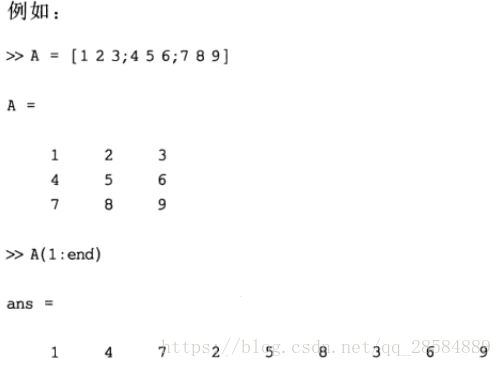两种方式的转换：

sub2ind函数

sub2ind is used to determine the equivalent single index corresponding to a given set of subscript values.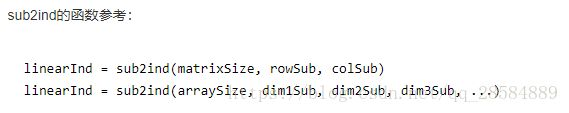二维矩阵： IND = sub2ind(SIZ,I,J)

SIZ是转换矩阵的大小，一般用size函数求得；

I是下标中的行数，J是下标中的列数；

返回值IND是转化后对应的序列号(索引号)；

ind2sub函数

ind2sub和sub2ind这对函数，是互逆的一对函数。ind2sub把数组或者矩阵的线性索引转化为相应的下标；sub2ind则正好相反，将下标转化为线性索引。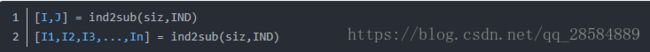展开全文matlab矩阵访问
• Matlab矩阵多元素的访问

千次阅读 2017-10-05 17:46:38
Matlab矩阵元素访问(1)A(a:b:c)表示取数组或矩阵A的第a个元素开始每隔b个步长一直到元素c (2)A([m n l])表示取数组或矩阵A中的第(m,n,l)个元素 (3)A(:,j)表示取A矩阵第j列的所有元素 (4)A(i,:)表示A矩阵第i行...matlab
• 我觉得应该有一个简单的解决方案,但我找不到它：我有稀疏矩阵A B具有相同的维度n * n.我想创建矩阵C,它复制A中的值,其中B不为零.这是我的方法：[r,c,v] = find(B);% now I'd like to create an array of values ...
• MATLAB矩阵的建立与访问 数值数据的输出格式（format） 一、 MATLAB 函数的调用格式 常用函数的应用 二、MATLAB矩阵表示及矩阵元素的引用 (1) 利用直接输入法建立矩阵 (2) 利用已建好的矩阵建立更大的矩阵 (3) 用...matlab python
• 文章原创，转载请注明出处MATLAB矩阵数组的下标是从1开始计数的而C语言或者C++之中则是从0开始的，而且MATLAB矩阵的单个索引值访问时是按列方向计数的，而C/C++中则是按行方向计数的，在考虑将MATLAB算法或者...
• 矩阵的乘法、乘方和除法有特殊的数学 含义, 并不是数组对应元素的运算, 所以......MATLAB矩阵与数组的区别,点运算符的运用一维数组相当于向量,二维数组相当于矩阵.所以矩阵是数组的子集 数组运算是指数组对应元素...
• 2.矩阵的寻访与赋值在创建了矩阵之后，我们经常需要访问矩阵中...1．全下标标识经典数学教科书在引述具体矩阵元素时，通常采用全下标标识法，即指出某一元素是在第几行第几列。这种标识方法的优点是：几何概念清楚，...
• 数组 - 如何在MATLAB中迭代n维矩阵中的每个元素？我有个问题。 我需要在MATLAB中遍历n维矩阵中的每个元素。 问题是，我不知道如何为任意数量的维度做这件事。 我知道我可以说for i = 1:size(m,1)for j = 1:size(m,2)...
• MATLAB利用双下标提取矩阵元素%利用双下标提取矩阵元素 format short r=randn(3) r1=r(1,1) %访问r（1，1）处的值 r2=r(2,3) %访问r（2，3）处的值 r3=r(3,3) %访问r（3，3）处的值 运行结果如下matlab
• MatLab矩阵

2021-08-23 10:05:50MATLAB 矩阵
• MATLAB矩阵的基本运算1.1 数值矩阵的生成1．实数值矩阵输入输入矩阵方法：用键盘上方括号“ [ ]”代替矩阵括号。矩阵数据在方括号内按行输入，同一行相邻元素用空格(或逗号)隔开，相邻两行用分号“；”隔开。 例如...
• MATLAB矩阵及其运算》由会员分享，可在线阅读，更多相关《MATLAB矩阵及其运算(10页珍藏版)》请在人人文库网上搜索。1、Matlab矩阵及其运算目录矩阵创建1矩阵的基本运算3矩阵的行列式运算3矩阵的求逆运算4矩阵的求...
• 数组元素的标识与寻访(作者：lcc)数组元素的标识n “全下标(index)”标识经典数学教科书采用“全下标”标识法：每一维对应一个下标。Ø 如对于二维数组，用“行下标和列下标”标识数组的元素，a(2,3)就表示二维数组a...
• matlab矩阵转置命令

千次阅读 2021-04-18 06:01:59
matlab矩阵的转置怎么表示matlab中， 矩阵A的转置表示成 A' 【附注】matlab中常用的矩阵运算矩阵加、减(＋,－)运算 规则： (1)相加、减的两矩阵必须有相同的行和列两矩阵对应元素相加减； (2)允许参与运算的两矩阵...
• 有流量的可以直接戳视频二狗在用matlab学习编程过程中，发现matlab中有大量矩阵运算，矩阵的知识了解不到位，在学习算法的过程中无法找到合适的解决问题的方法或者出现编程错误。好比英语发音规则都不懂，...
• matlab提取矩阵第一列

千次阅读 2021-04-29 09:44:59
第2章 MATLAB矩阵及其运算 2.1 变量和数据操作 2.2 MATLAB矩阵 2.3 MATLAB运算 2.4 矩阵分析 2.5 矩阵的超越函数 2.6 字符串 2.7 结构数据和单元数据 2.8 稀疏矩阵 2.1 变量和数据操作 2.1.1 变量与赋值 1....
• 矩阵的创建一般可以使用以下三种方法：第一种是直接输入矩阵元素；第二种是通过调用函数生成特殊矩阵；第三种是利用M文件创建矩阵，矩阵的访问则有下标法、索引法等方法。如我们通过第一种方法创建三个矩阵，他们...
• 我觉得应该有一个简单的解决方案,但我找不到它：我有稀疏矩阵A B具有相同的维度n * n.我想创建矩阵C,它复制A中的值,其中B不为零.这是我的方法：[r,c,v] = find(B);% now I'd like to create an array of values ...
• MATLAB矩阵分析

2017-06-29 09:54:17
一、矩阵的基础知识 close all; clear all; clc; %% 改变矩阵尺寸 a=eye(3);...%添加第四列，第二行元素为3，其余为0 ...%将矩阵变为行向量，以列为顺序，end表示最后一个元素 %% 改变矩阵形状 a=magiMATLAB 矩阵分析
• matlab中文名是矩阵实验室，由此可知matlab主要是基于矩阵进行的一系列操作，本文主要讲述一些有关数组和矩阵的创建、访问和运算的一些最基本的操作，帮助我们对matlab有一个直观的理解，同时可以借助matlab完成一些...
• 相关问题:匿名网友:matlab中取一个矩阵的一部分方法如下：格式A(m,n)，用于提取矩阵A中符合m,n要求的部分1、提取某个元素，则m,n为数字标量，如A(2,3)为第二行第三列的元素。2、提取某行某列A(：，n)提取第...
• 慕标5265247这类问题是反复出现的，应该比“Matlab使用高度优化的库”或“Matlab使用MKL”一次更清楚地回答堆栈溢出。历史：矩阵乘法(与矩阵向量、向量乘法和许多矩阵分解一起)是线性代数中最重要的问题。工程师们从...
• 使用bsxfun创建正确的索引...这段代码的作用是创建一个2D矩阵,其中每一行都是每个感兴趣的窗口访问元素.如果你想直观了解代码如何生成索引,请特别注意第一种情况,即X = 1;和W = 3;我们可以看到第一行包括访问元素1......

matlab矩阵元素访问matlab 订阅